## Monday, April 18, 2011

I've been off -the-air a bit more this week as I implement some upgrades to my computing capability.

I now have my desktop & laptop Macs running 10.6 (Snow Leopard) and installing as much software as I can to run 64-bit mode.  This will make it easier to handle some of the larger datasets required for this project.  I addition, I hope to mirror the working directories between the desktop & laptop systems, adding an extra level to my regular backups.

I'm making the page describing the installation configuration of my scientific tools available here, both for my future reference and in the event others wish to duplicate my setup.  Plus, I have repeatedly made use of the descriptions others have placed online and this is just my way to contribute and return the favor.

This project should be complete soon and I can return to more regular posting.

## Saturday, April 9, 2011

### Geocentrism: Does NASA use Geocentrism?

Here's the rest of my response to James Phillips, from his comment:

“Is it true that N.A.S.A. uses the geocentric model rather than the heliocentric model and if so what is their rationale for doing so.“

This is a very poorly worded question.  The short answer is, “Of course they do - where appropriate.”

They use longitude, latitude, and altitude when it is the convenient unit of measure - and they use these quantities on the Earth, Moon, Mars, etc.  Why would they not?  Why would you reference everything with heliocentric coordinates if you are in orbit around the Earth?  Or in orbit around Mars?  The heliocentric coordinates are just a coordinate transformation away from any other coordinate system you choose to use.

You use the model appropriate to the scale of the problem you are solving. A geocentric model can be sufficiently accurate near the Earth, but deviates as one moves further away from the Earth.  When traveling between planets, NASA routinely transitions between the frame of the Earth, to the heliocentric frame, and to the frame of the target planet when the spacecraft gets near.

NASA also has datasets where the Earth is treated as FLAT.  I'm sure the Flat Earthers will regard that as vindication for their interpretation of Scripture (Wikipedia, Flat Earth Society).

Near Earth

For near Earth trajectories, the coordinate system of choice is GEI (Geocentric Earth Inertial) which is fixed with respect to the distant stars. This is the one you use to compute your trajectories as Newton's laws and gravity apply in their simplest form.

If you want to know where your satellite is visible from the surface of the Earth, you use GEO (Geocentric body-fixed) which rotates with respect to GEI.   It is simple to convert between these two systems (unless you want to include nutation), just a rotation around the z-axis that completes one rotation in a sidereal day.   This coordinate system is often used for transferring data to and from tracking stations.  Other coordinate systems I've used in my day job are described here: Coordinate systems and transformations, GEOPHYSICAL COORDINATE TRANSFORMATIONS.

There are similar planetocentric coordinate systems used for close flybys and orbits by spacecraft.

Since there are a number of spacecraft at Mars, which coordinate system do you think they use for tracking spacecraft?  See MSL Update to Mars Coordinate Frame Deﬁnitions (2006), pg 6:
“When a spacecraft is in the vicinity of Mars, it is convenient to utilize Mars-centered coordinate systems. These are systems that are centered at the center of the planet itself, as opposed to the system barycenter or on the planet surface. The systems described here are utilized regularly by the ﬂight operations and mission planning teams for JPL Mars missions.”
Beyond the Earth

If you want to compute trajectories farther from the Earth, say to go to Venus, or Mars, or beyond, you compute the trajectories in a heliocentric system (or more accurately a heliocentric barycenter system), again because the laws of motion and gravity apply in their simplest form, which means you can compute future (or past) positions more accurately. There are two methods for doing this.
1. Compute planetary positions as a full N-body simulation (Scholarpedia).
2. There are algorithms which start with a reference elliptical orbit (heliocentric barycenter) and then compute how the gravitational forces from the other planets perturb that orbit. These perturbations show up as slow variations in the orbital parameters (called Secular variations of the planetary orbits, or VSOP). From that, you install your spacecraft position and velocity, subject to the same laws of motion and gravity. Once you know the position of your spacecraft in the heliocentric frame, you can compute where the object would appear from any other location by using a coordinate transformation, such as those described above. You would convert to the GEO system if you wanted to know where to point an Earth-based antenna to send commands to your spacecraft or receive data.
Check out JPL: Basics of Space Flight: Interplanetary Trajectories.  The web tool JPL: Solar System Dynamics: HORIZONS Web-Interface computes trajectories of many solar system objects and can transform the positions as they would appear at other locations in the solar system. The FAQ on the Horizons system also has info on computing planetary positions which I have utilized.

Orbital dynamics is so precise, we can compute trajectories decades before an actual launch. We can compute if existing boosters have the capability to send a spacecraft onto a given trajectory. If we need a new booster to handle more distance, higher speed, or more payload, we can compute those requirements before we cut a single piece of metal to build it. We don't build the biggest rocket we can, fuel it up, and hope it makes it to the destination. How is that done? (For those who want to bring up the Pioneer Anomaly (Wikipedia), it is looking more and more like this is not new physics, but a very tiny thrust created by emission of heat from the spacecraft).

Strange Way to Run a Cover-up...

This is not just a NASA thing.  ESA, Japan, India, China and other countries are sending spacecraft to other planets. Are they part of the coverup as well? All the data and mathematics for computing interplanetary trajectories are a matter of PUBLIC record. Many of these techniques were developed over 100 years before NASA even existed. Amateur astronomers who understand the math can do these computations on their desktop computers to far higher accuracy than those researchers from the 1700s to the 1950s who developed the techniques via hand calculation, slide-rule, and adding machine. Today, this is a project of college undergraduates (see Interplanetary Trajectory Development). I ran simple solar system models on an N-body code I wrote on an Apple II(Wikipedia) back in 1980 while an undergraduate.

With so many people who have the knowledge of how to do this, it's a strange way to run a 'conspiracy' against Geocentrism (Moving-World DECEPTION).

The Real Conspiracy?

Perhaps the more interesting question would be what computations are the supporters of Geocentrism Galileo was Wrong using when they do their graphics? How are they computing the position of, say Jupiter, in the sky on a given date and to what accuracy? Can they compute when the ISS will pass over my location? Are their graphics constructed using software where the computations are in a heliocentric system or are they doing the computations themselves in a geocentric system?

If they're doing the computations themselves, why don't they show their work so that others can use (and test) them as well?

Here's the NASA info on trajectory and navigation for spacecraft:
Here's some work by people OUTSIDE of NASA
Some months ago, Rick DeLano claimed that Geocentrism could explain the Lagrange points, five points of stability in the restricted 3-body problem (Wikipedia), and one of the predictions of Newtonian gravity and laws of motion. I challenged him to demonstrate it, considering that we make use of these locations in a number of operating space missions.
The Geocentrists have been strangely silent on this.

If you taught Geocentrism in a physics class, how would you use this knowledge to plan spacecraft missions?

Here's a syllabus of an astrodynamics class at Georgia Tech.  This is training for people to really do this work.  I wonder if any of the Geocentrists could do the homework problems posted here.  How would they answer the practical problems of interplanetary navigation in a Geocentric model?  As yet, Geocentrists have not demonstrated any competence in this field where they claim so much knowledge.  Unless you believe all spaceflight is a hoax (or you chicken out and just claim everything beyond Earth orbit is a hoax), your only other choice with Geocentrism is to terminate all space flight, leaving space travel to other countries less entrenched in dogma.

These are not idle questions of only philosophical interest.  Billions of dollars in space assets, the lives of astronauts, even national security, rely on doing this stuff right.  Would you trust these things to those who have not demonstrated any competence in the topic?

## Sunday, April 3, 2011

### Quantized Redshifts. X. Testing Our "Designer Universe"

In the last section, I demonstrated, by a simple exercise, how scientists can make model datasets to test their analysis methods.  Of course, once we build these test datasets, we should always conduct some tests on the results to make sure they exhibit the properties we seek for our testing.  That is the purpose of this section.

Consider yourself at the center of a uniform distribution of galaxies (homogeneous), all around you, covering the entire sky (isotropic).  We'll assume they have a constant mean density (number of galaxies per cubic megaparsec) out to the edge of the distribution (homogeneous).  If we have a spherical sampling of galaxies, with a uniform number density, n (galaxies per unit volume), it is easy to compute the number of galaxies per bin, dN, that must be in a thin spherical shell at distance, r, and thickness, dr.

dN = n 4 \pi r^2 dr

This is just the area of the sphere, multiplied by the thickness (to give a volume) all multiplied by the density.

We can compute this function for our array of redshift values using the histogram function.  First we create an array of nbins bins in redshift space (zbinning) out to a maximum value of z, maxz.

zbinning=np.arange(0.0,maxz,maxz/nbins)
distribution=np.histogram(redshift,zbinning)

I plot the result for one of the model runs below with maxz=0.512 and nbins=512.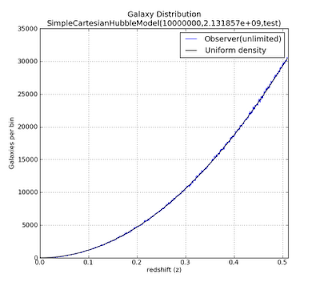Figure 1: Click to enlarge

The blue, slightly irregular, line represents the number of galaxies in each bin of redshift values.  The irregularities are due to the statistical variation in the model.  The black line is an analytic curve of the density distribution.  We see that the two curves have excellent agreement, with the blue curve exhibiting slight statistical fluctuations around the expected count given by the black curve.

This is the kind of distribution of galaxies with redshift, z, that we would expect to observe if we were in a VERY large, VERY uniform universe, and our telescopes have infinite sensitivity, i.e. we see all galaxies no matter how faint.  This is a very unrealistic assumption, and we we will deal with making it more realistic soon.

As an additional test, we can use our distribution counts to compute the density, n, of each radial shell,

n = dN / ( 4 \pi r^2 dr)

We plot the results below.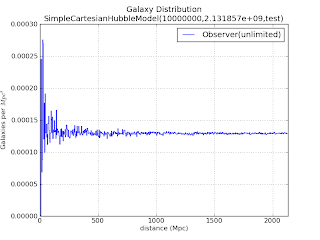Figure 2: click to enlarge

We see that at large distances, the value of n exhibits small fluctuations around the mean density of the input model.  That mean density is computed by dividing the total number of galaxies by the total volume of our model cosmos.  As we sample distances closer to the observer, the fluctuations get larger and larger.  This is not unexpected.  At smaller radii, the number of galaxies in the bin gets smaller and this this makes the statistical error in each bin is larger since it can only have an integer number of galaxies in a bin (no fractional galaxies).   Our input galaxy density is actually less than 1 galaxy per Mpc^3, so when we sample some bins near the center, the probability of having bins with much less (or more) than the mean number of galaxies can show large fluctuations.  After all, at these bin sizes, we can only have integer numbers of galaxies in the bins, either zero or one, but rarely more.

Again, the model behaves as we expect, consistent with our input assumptions.

Making Our Designer Universe More Realistic

But in reality, we do not have telescopes of infinite sensitivity.  To simulate the effect of more realistic telescopes, we will assume the telescopes can only see galaxies brighter than a given limiting apparent magnitude, limitingMagnitude.  For this experiment, we'll choose a limiting magnitude of 18 and 20.  We can plot the distribution of our galaxies in distance vs. apparent magnitude.

First we find the array indices of galaxies in our model catalog that are less than  limitingMagnitude:
magLimitIndices=np.nonzero(apparentMagnitude < limitingMagnitude)

With this array of indices, we can then select out the galaxies from our source arrays, distance, apparentMagnitude, making new arrays, of the galaxies that fall in our magnitude limits.

distances = np.take(distance,magLimitIndices)

apparentMagnitudes = np.take(apparentMagnitude,magLimitIndices)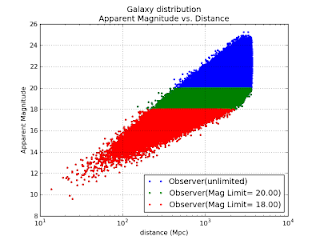Figure 3: click to enlarge

In this plot, the red markers represent all galaxies brighter than magnitude 18.0.  The green band reveals galaxies fainter than magnitude 18 but brighter than magnitude 20, and blue are the remaining galaxies in our catalog.  Note that the distance is a logarithmic scale.  Apparent magnitude is a logarithmic rescaling of energy flux.

At small distances, we count very few galaxies.  This is why our mean density fluctuated so wildly in the bins for near-Earth distances.  Your sampling either caught some galaxies, or it didn't.  The further out we go, we see more galaxies along the vertical line of a fixed distance, up until the point our survey starts missing galaxies because they are too faint.  At this point, the number of galaxies begins to decline until we reach a distance so great that no galaxy is bright enough to be detected.

How that would look in a histogram?

We can compute the mean density again, as above, but this time plotting the result for each different limiting magnitude.  We plot this result below.

distribution=np.histogram(distances,rbinning)
# convert to galaxy density/Mpc^3
density=distribution*1e18/(rbinning[:-1]*rbinning[:-1])/(boundary/nbins)/(4.0*np.pi)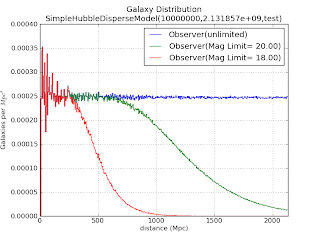Figure 4: click to enlarge

As before, we have large fluctuations in mean density near the Earth, which decreases as the distance from the Earth increases.  But now we have the additional effect of our limiting magnitude of detection.  At some distance, the measured density begins to decrease, as our number of galaxies measured at that distance begins to decrease.  The red, green, and blue curves in Figure 4 represent the corresponding model color in Figure 3.

Food for Thought:  In this exercise, I've examined the case of a survey limited by telescope sensitivity.  Suppose we had telescopes that had a much higher magnitude limit, so our survey probed so deep into the cosmos that we saw fewer galaxies because we were sampling a time when galaxies were just forming?

Now we need to sample this dataset constructed in 3-dimensions, into a radially-sampled dataset as done in the first figure of this article.  We also compute this histogram for our experimental magnitude-limited surveys.

For each of the subsamples above, we can again compute the histogram in redshift-space.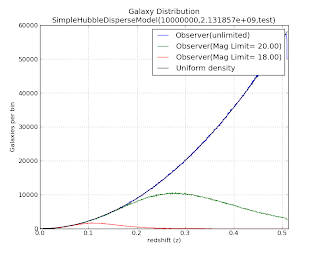Figure 5: click to enlarge

The black curve is the radial galaxy count profile one expects for a uniform density.  The green curve corresponds to the distribution with a limiting magnitude of 20, and the red curve corresponds to a distribution with a limiting magnitude of 18.

Let's zoom in on the red curve, expanding the vertical scale.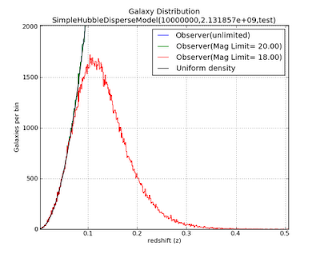Figure 6: click to enlarge

If you've examined a data from a number of large-scale sky surveys, the profile of the red curve might look a bit familiar.

Next (but probably NOT as soon as next weekend): What Can We Learn from this Model and Questions for Further Exploration